# ABCD Matrix

ABCD Matrixesare used in paraxial optical design.

It’s only allowed to use ABCD Matrices in the paraxial range, withThe angles are measured in radians!

A beam is described by a distance r from the optical axis and a offset angle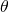from the optical axis
An ABCD Matrix that describes the optical element is formed
The ABCD Matrix is multiplied by the input vector
The result is an output vector that describes the output beam with a new distance from the optical axis and a new angle off the optical axisis a short for for the equation systemwithwith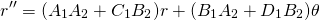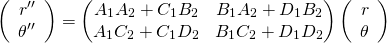Examples of ABCD matrices for simple optical elements :

Propagation in free space or in a medium of constant refractive index :Where d = reduced distance= thickness / refraction index
Propagation through a series i=1..k of planparallel media with constant refraction indices: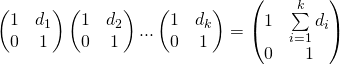Where d_i = reduced distance_i= thickness_i / refraction index_i

Refraction at a flat surface:Where:= initial refractive index= final refractive index
Refraction at a curved surface:Where:
R = radius of curvature,for a convex surface (centre of curvature after interface)= initial refractive index= final refractive index
Reflection vrom a flat mirror:Identity matrix
Reflection from a curved mirror:Where:effective radius of curvature in tangential plane (horizontal direction)effective radius of curvature in the sagittal plane (vertical direction)
Withfor convex mirrors (centre of curvature after interface)
Refraction at a thin lens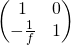Where:
f = focal length of the lens, wherefor convex/positive/converging lenses. Valid if if and only if the focal length is much bigger than the thickness of the lens
Refraction at a thick lensWhere:= refractive index outside of the lens.= refractive index of the lens itself (inside the lens).= Radius of curvature of First surface.= Radius of curvature of Second surface.
t = center thickness of lens.